The Structure and Representation of Organic Molecules

The Structure and Representation of Organic Molecules

Introduction

When chemicals bond, they can form complicated three-dimensional shapes. This article will discuss how to represent molecules and how to understand and predict their structure in three dimensions.

Key Terms

• Molecular formula
•  Isomer
• Structural formula
• Formal charge
• Resonance
• Localized (electrons)
• Delocalized (electrons)
• Valence shell electron pair repulsion (VSEPR)

Objectives

• Represent organic molecules using clear, simple drawing techniques
• Understand the role of resonance and electron delocalization in certain molecules
• Determine molecular structure using the VSEPR model
Drawing Molecules

Drawing Lewis structures, including all the nonbonding electron pairs, can be a tedious exercise, especially when we are dealing with large molecules. We will generally omit depicting nonbonding electron pairs-but those electron pairs are still there, and they have an effect on molecular structure, as we will discuss later. Even omitting these electron pairs, however, still leaves complicated diagrams in some instances. Consider the following molecule: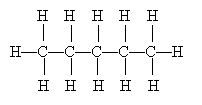Clearly, this type of diagram can quickly become very crowded and even quite confusing. Because organic chemistry deals with carbon, such diagrams are often drawn without showing the 'C' to represent carbon. Furthermore, the hydrogen atoms (protons, essentially) are also omitted on the assumption that they represent the remainder of the needed covalent bonds to satisfy the octet rule for carbon. Thus, the diagram above can be drawn simply as the following, where the carbon atoms are at the endpoints of the line segments: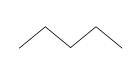When other atoms, such as oxygen and nitrogen, are included in a molecule, these are shown explicitly, as are any hydrogen atoms bonded to these elements. Thus, for example, we might draw the following molecule: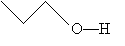Or, more simply,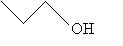Structural Formulas

The first molecule we drew above contains five carbon atoms and 12 hydrogen atoms; thus, we might write the molecular formula as C5H12. But this isn't always helpful, as each of the following arrangements of these atoms satisfies this formula.These different arrangements are called isomers, because they all have the same molecular formula but differ in their structures. To differentiate isomers when writing molecular formulas, we can write the formula in terms of the structure of the molecule (a structural formula) by noting how different groups are attached to each atom.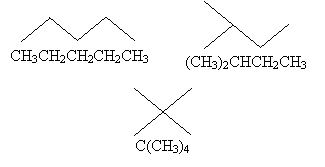Practice Problem: What are the molecular formulas for the three molecules drawn below?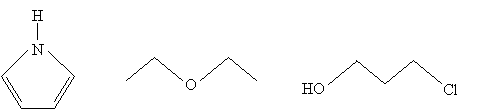Solution: One way to make finding the molecular formula easier in each case is to draw all the hydrogen atoms that are implicit in the above diagrams. Remember that the endpoints of the line segments, assuming they don't end at another atom, represent carbon atoms. Check each atom to be sure it obeys the octet rule. Count the number of each type of atom, then write the formula.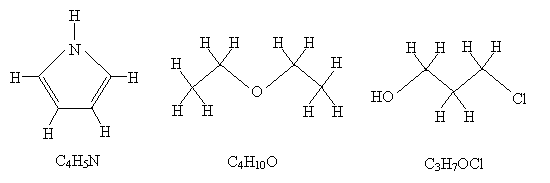Practice Problem: Draw diagrams and write structural formulas for the (neutral) isomers of C4H10

Solution: Determining isomers for a given molecule is something of a matter of trial and error. In each case, however, be sure that the octet rule is satisfied and that the molecule contains the proper numbers of each atom (four carbons and 10 hydrogens).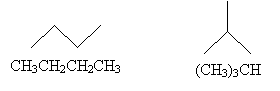Resonance

The ozone molecule has a molecular formula O3. The following is a Lewis structure representing ozone.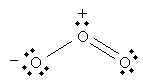Note that each oxygen atom has a full valence shell, but one oxygen atom is marked with the negative sign (-) and one is marked with a positive sign (+). These signs indicate that these atoms have a net charge, but if we count the total number of valence electrons in the molecule (18), we find that the entire molecule is neutral (each oxygen has six protons). The charge on the individual atoms is its formal charge. To calculate the formal charge for an atom, subtract its electron count (the number of electrons in nonbonding pairs plus half the number of electrons in covalent bonds for that atom) from it's the number of valence electrons associated with the neutral atom. In the above ozone diagram, the formal charge of the leftmost oxygen atom is calculated as follows: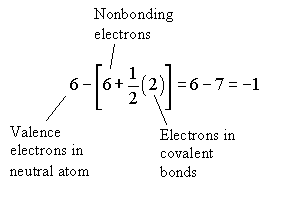But the following is also a legitimate Lewis structure for ozone.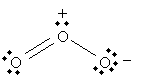In fact, both of these structures are legitimate; we can, in some sense, view ozone as "alternating" between these two structures: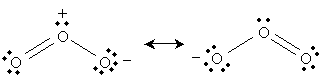This resonance is (described simplistically using Lewis structures) the result of a nonbonding electron pair on the negatively charged oxygen becoming a covalent bond with the central oxygen, pushing a covalent bond to the third oxygen into a nonbonding electron pair. We can show these actions using curved arrows: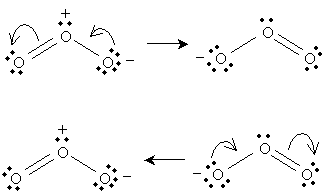However, electrons are not particles in the sense indicated by the Lewis structures: they do not have definite locations (i.e., they are not localized to a particular atom), but probabilities of being located in a particular place (that is, they are delocalized). Thus, a more accurate way of depicting "resonance" (which is not really resonance in the common sense of the word) is to show the central oxygen as having one and one-half covalent bonds with each peripheral oxygen: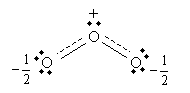Thus, one pair of electrons is delocalized among the central and peripheral oxygen atoms, forming a "half" covalent bond on either side. This is closer to the quantum mechanical model of electron behavior in this case (as well as closer to experimental results).

Practice Problem: Nitric acid (HNO3, whose structural formula can be written (OH)NO2) has two primary resonance forms. Draw the Lewis structures for these forms.

Solution: Use the structural formula to guide you in drawing the molecule. Then, apply the octet rule to determine the locations of the bonds and nonbonding electron pairs.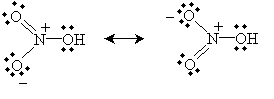Alternatively, you can draw the equivalent "half-bond" representation: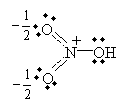VSEPR and Molecular Structure

Although the limits of pen and paper limit us to two-dimensional drawings of molecules, in reality, they have three-dimensional configurations. A guiding principle that can provide a qualitative guide for determining the spatial arrangement of atoms in a molecule is the valence shell electron pair repulsion (VSEPR, pronounced "vesper") model, which states that an atom's electron pairs (whether bonding or nonbonding pairs) will seek to be as far from the other electron pairs as possible. In the simple case of methane (CH4), for instance, the hydrogen atoms will form a tetrahedral arrangement around the carbon atom.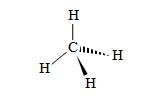In this method of drawing molecules, the standard lines indicate covalent bonds in the plane of the page. Solid wedges indicate bonds that come up (to some extent) out of the plane of the page, and hashed wedges indicate bonds that go down (to some extent) into the plane of the page. Note that this tetrahedral arrangement, the bonding electron pairs are as far from all the other pairs as possible.

Nonbonding electron pairs, because they are not shared between two atoms, repel each other more strongly than do electron pairs that form a covalent bond. Thus, for instance, the angle formed between the hydrogen atoms in water (H2O) is smaller than the angle formed between the oxygen's two nonbonding electron pairs (shown below as orbital "lobes" for the purposes of illustration). The angles in the diagram are not to scale, but they illustrate that the nonbonding electron "lobes" tend to repel each other more than do the bonding (shared) electron pairs. The arrangement of water below is called bent.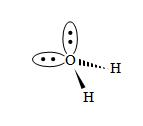Practice Problem: Use the VSEPR model to sketch a three-dimensional representation of ammonia (NH3).

Solution: The nitrogen in ammonia has three covalent bonds (one to each of three hydrogen atoms) and one nonbonding electron pair. These four electron pairs will seek to be as far from each other as possible, with the nonbonding pair repelling the bonding pairs a little more than the bonding pairs do each other.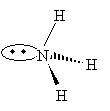Note that the molecule (ignoring the nonbonding pair) is shaped like a pyramid-this geometrical arrangement is called trigonal pyramidal